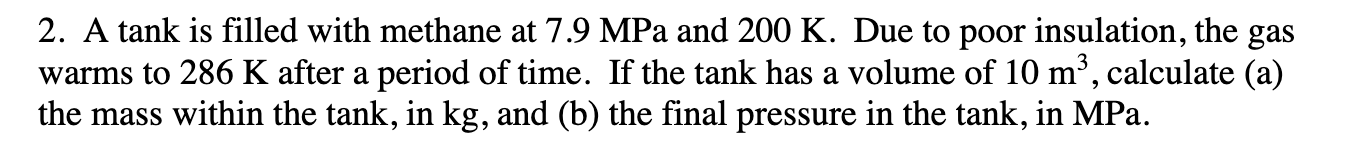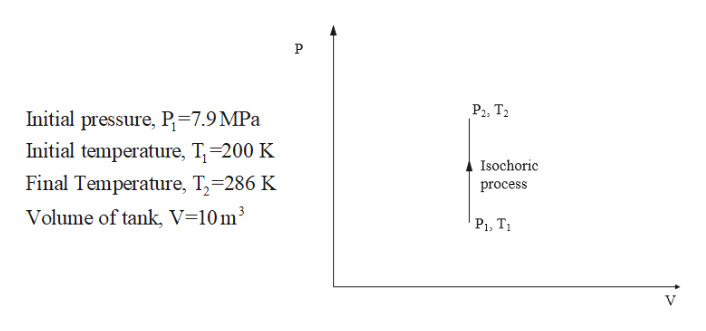# 2. A tank is filled with methane at 7.9 MPa and 200 K. Due to poor insulation, the gaswarms to 286 K after a period of time. If the tank has a volume of 10 m2, calculate (a)the mass within the tank, in kg, and (b) the final pressure in the tank, in MPa

Question
25 viewshelp_outlineImage Transcriptionclose2. A tank is filled with methane at 7.9 MPa and 200 K. Due to poor insulation, the gas warms to 286 K after a period of time. If the tank has a volume of 10 m2, calculate (a) the mass within the tank, in kg, and (b) the final pressure in the tank, in MPa fullscreen
check_circle

Step 1

Given:help_outlineImage TranscriptioncloseP Р, Т. Initial pressure, P=7.9 MPa Initial temperature, T=200 K Isochoric Final Temperature, T-286 K process Volume of tank, V=10 m3 Р, Т. V fullscreen
Step 2

Assumptions

1. It is assumed that methane is an ideal gas.
2. Specific heat is constant.

The methane is gaining heat at constant volume thus it is an isochoric heat addition process as shown in the figure of step 1.

The value of gas constant R for methane is taken from the thermodynamic property table

Step 3

a

Apply ideal gas law ...

### Want to see the full answer?

See Solution

#### Want to see this answer and more?

Solutions are written by subject experts who are available 24/7. Questions are typically answered within 1 hour.*

See Solution
*Response times may vary by subject and question.
Tagged in

### Thermodynamics